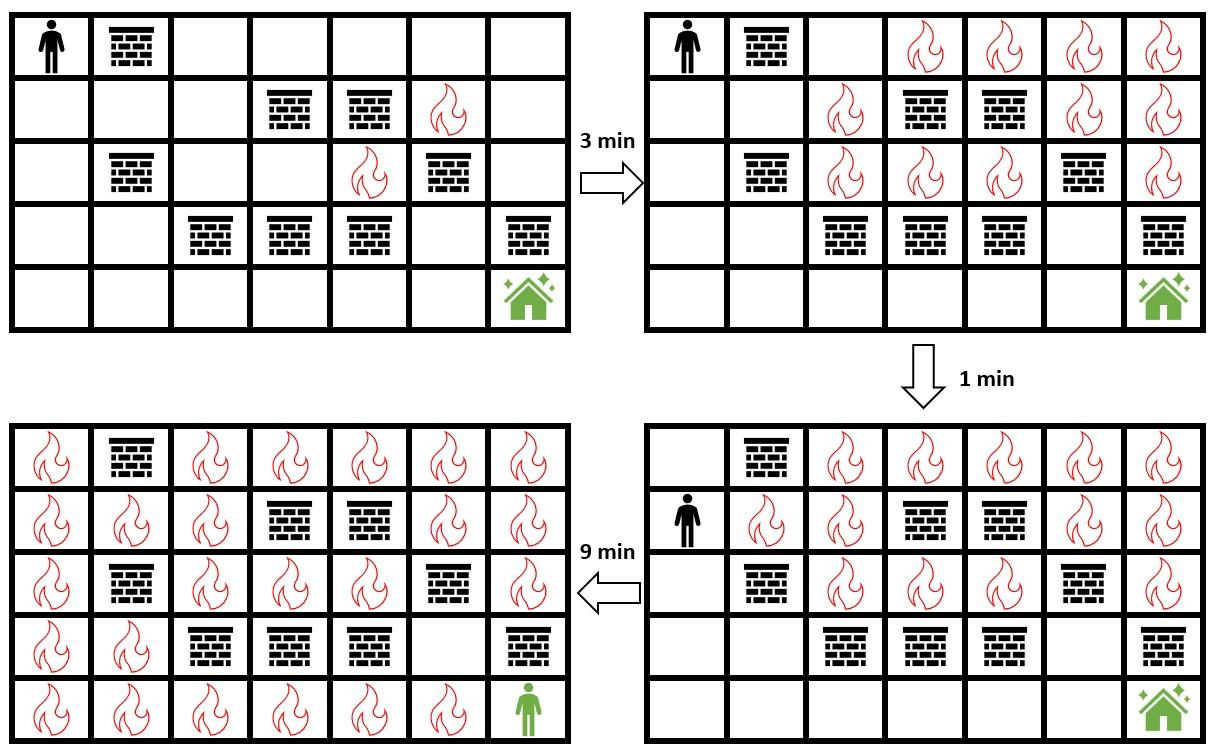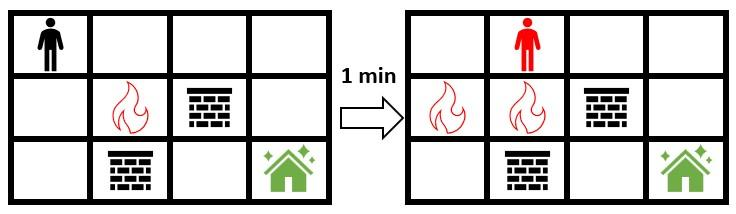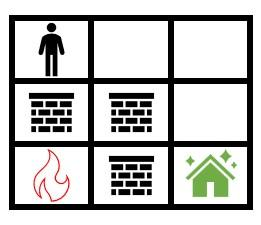# leetcode 2258. Escape the Spreading Fire（python）

### 描述

You are given a 0-indexed 2D integer array grid of size m x n which represents a field. Each cell has one of three values:

• 0 represents grass,
• 1 represents fire,
• 2 represents a wall that you and fire cannot pass through.

You are situated in the top-left cell, (0, 0), and you want to travel to the safehouse at the bottom-right cell, (m - 1, n - 1). Every minute, you may move to an adjacent grass cell. After your move, every fire cell will spread to all adjacent cells that are not walls.

Return the maximum number of minutes that you can stay in your initial position before moving while still safely reaching the safehouse. If this is impossible, return -1. If you can always reach the safehouse regardless of the minutes stayed, return 109.

Note that even if the fire spreads to the safehouse immediately after you have reached it, it will be counted as safely reaching the safehouse.

A cell is adjacent to another cell if the former is directly north, east, south, or west of the latter (i.e., their sides are touching).

Example 1:``````Input: grid = [[0,2,0,0,0,0,0],[0,0,0,2,2,1,0],[0,2,0,0,1,2,0],[0,0,2,2,2,0,2],[0,0,0,0,0,0,0]]
Output: 3
Explanation: The figure above shows the scenario where you stay in the initial position for 3 minutes.
You will still be able to safely reach the safehouse.
Staying for more than 3 minutes will not allow you to safely reach the safehouse.
``````

Example 2:``````Input: grid = [[0,0,0,0],[0,1,2,0],[0,2,0,0]]
Output: -1
Explanation: The figure above shows the scenario where you immediately move towards the safehouse.
Fire will spread to any cell you move towards and it is impossible to safely reach the safehouse.
Thus, -1 is returned.
``````

Example 3:``````Input: grid = [[0,0,0],[2,2,0],[1,2,0]]
Output: 1000000000
Explanation: The figure above shows the initial grid.
Notice that the fire is contained by walls and you will always be able to safely reach the safehouse.
Thus, 109 is returned.
``````

Note:

``````m == grid.length
n == grid[i].length
2 <= m, n <= 300
4 <= m * n <= 2 * 10^4
grid[i][j] is either 0, 1, or 2.
grid == grid[m - 1][n - 1] == 0
``````

• 0 代表草
• 1 代表火
• 2 代表不能穿過的牆

### 解答

``````class Solution(object):
def maximumMinutes(self, grid):
"""
:type grid: List[List[int]]
:rtype: int
"""
M, N = len(grid), len(grid)
timeArrive = [[float("inf") for _ in range(N)] for _ in range(M)]
fire = []
for i in range(M):
for j in range(N):
if grid[i][j] == 1:
fire.append([i, j])
timeArrive[i][j] = 0
stack = []
for i, j in fire:
stack.append((i, j, 0))
while stack:
x, y, time = stack.pop(0)
for i, j in [[x + 1, y], [x, y + 1], [x, y - 1], [x - 1, y]]:
if 0 <= i < M and 0 <= j < N and grid[i][j] == 0 and timeArrive[i][j] > time + 1:
timeArrive[i][j] = time + 1
stack.append((i, j, time + 1))

def waitK(k):
h = [(0, 0, k)]
vis = set()
while h:
x, y, time = h.pop(0)
for i, j in [[x + 1, y], [x, y + 1], [x, y - 1], [x - 1, y]]:
if i == M - 1 and j == N - 1 and timeArrive[i][j] >= time + 1:
return True
if 0 <= i < M and 0 <= j < N and grid[i][j] == 0 and timeArrive[i][j] > time + 1 and (i, j) not in vis:
h.append((i, j, time + 1))
return False

if waitK(2*10**4):
return 10**9
elif not waitK(0):
return -1
l,r = 0, 2*10**4
while l<r:
mid = (l+r)//2
if waitK(mid):
if not waitK(mid+1):
return mid
else:
l = mid + 1
else:
r = mid
return l
``````

### 執行結果

``````55 / 55 test cases passed.
Status: Accepted
Runtime: 1033 ms
Memory Usage: 16.2 MB
``````